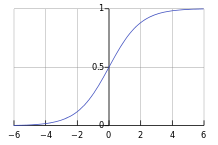No function is an island (okay; some might be, but still..). Functions work with one another to achieve a goal. Let’s take a simple example of finding the third element of a list:

The deep nesting of function calls might come across as an eye-sore. Enter function composition, a teeny-tiny treasure hidden in your high-school algebra textbook:

The comp function

Clojure standard library provides a comp function, that takes in a bunch of functions and returns a composition of those functions. When applied to a set of arguments, the inner-most function consumes the arguments first and passes on its results to the next function in the chain: eventually the outer-most function executes, yieliding the final result.

As an example, (comp f g h) would return a composition function. When a set of arguments, say x y are applied to the composition function:

• h evaluates on x y first
• The result obtained from above is passed to g
• The result obtained from above is passed to f
• The result obtained from above becomes the output of the composition function

Rewriting our third function this way gives us a function that has fewer brackets, and arguably a bit more readable:

Eta-reduction

We can go one step further and use eta-reduction. To exemplify, instead of saying $k(x) = (f \circ g \circ h)x$, we could just say $k = f \circ g \circ h$, effectively ‘cancelling’ out x from the left-side and right-side. This leads us to an even better way of writing third:

It may be interesting to note that we used def here, and not defn, as there are no formal arguments to declare up-front. This is equivalent of proudly proclaiming “third is a composition of first, rest and rest functions; in that order”.

Example: sigmoid function

The sigmoid function is widely used in Machine learning classification problems, where we want to map a real number z to the interval (0, 1). The function definition is:

The plot looks like:To break-down the problem, we could conceptually write the sigmoid function as:

Leveraging function composition and eta-reduction,

So how does it look like in Clojure?

Two functions are out-of-the-box: minus is - and oneplus is inc. There is no standard reciprocal function: however it is nothing but a curried divide function where the numerator is 1. In other words, it is (partial / 1). Ditto for the epow function, which is a curried exponent function where the base is the constant 2.7182.

Let’s put all this together:

Can I compose everything in the world?

Of course not. Functions need to follow a chain (where output of one feeds as input to another) so that we can compose them. Not all functions follow this pattern. Take for instance the quadratic solution function having multiple parameters (a, b and c), which are referenced all over the place:

Nevertheless, one should watch out for sub-parts of functions where composition can be utilized. Take for example the Pythagorean formula to compute the length of the hypotenuse:

In Clojure, we could write the function as:

In the process of finding the root of sum of squares, we could still abstract out root of sum as a composition i.e. (comp math/sqrt +).

Javascript: an honourable mention

A discussion on a functional programming concept cannot be complete without mentioning javascript. There is no standard function to perform composition, and is easy to cook up one:

Briefly speaking, comp takes in a bunch of functions and returns one function. This function takes in some parameters which is directly fed to the inner-most function. Observe that the fns are reversed, because function application is right-to-left.